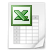### Each magic sum

A pure magic (4x4) square with all the numbers from 1 up to n x n (1 up to 16) has one fixed magic sum ([1+n x n]/2 x n), for example ([1 + 4 x 4]/2 x 4) = 34. You can skip the boundary of the pure magic square. In a not pure magic square all numbers must be different, but it don't need to be consecutive numbers. If the magic square is not pure it is possible to choose a magic sum. If you choose the right numbers to fill in the magic square you get the magic sum you want. See below an impure 4x4 magic square with the magic sum of 0.

 22 14 -46 10 -30 -6 6 30 26 2 -2 -26 -18 -10 42 -14

To get the magic sum of 403, divide 403 by 4 and you get 100 remainder 3.

Add 100 to each cell and add the remainder of 3 extra to each yellow marked cell.

 125 114 54 110 70 94 109 130 126 102 98 77 82 93 142 86

This square is not panmagic, but has extra magic features (for example 2x2 sub-squares, horizontally swinging and vertical "ears"). Discover it yourself.

See below the mathematical alternative.

 a c+3 d+1 b+2 d+2 b+1 a+3 c b+3 d c+2 a+1 c+1 a+2 b d+3

a + b + c + d = magic sum -/- 6

Boundaries are: b - a >= 4 and c - b >= 4 and d - c >= 4 (to get always 16 different numbers in the magic square)

Choose the magic sum, for example 100. Take care that a + b + c + d = 100 - 6 = 94 (and a, b, c and d must differ minimum 4), for example:

 a = 7 b = 20 c = 31 d = 36

 7 34 37 22 38 21 10 31 23 36 33 8 32 9 20 39

Notify that this square is "Dürer" magic (it has the same magic features as the Dürer magic square).

The idea of Aridwana from Indonesia is to convert names into numbers, using the table below.

 A 1 B 2 C 3 D 4 E 5 F 6 G 7 H 8 I 9 J 10 K 20 L 30 M 40 N 50 O 60 P 70 Q 80 R 90 S 100 T 200 U 300 V 400 W 500 X 600 Y 700 Z 800

For example I have converted the names of myself, my wife and my two daughters:

Arie = A + R + I + E = 1 + 90 + 9 + 5 = 105
Conny = C + O + N + N + Y = 3 + 60 + 50 + 50 + 700 = 863
Iris = I + R + I + S = 9 + 90 + 9 + 100 = 208
Tamar = T + A + M + A + R = 200 + 1 + 40 + 1 + 90 = 332

4 x 4 family Breedijk magic square

 Arie 335 864 210 865 209 108 Tamar 211 Conny 334 106 333 107 Iris 866 1514 1514 1514 1514 1514 1514 1514 105 335 864 210 1514 865 209 108 332 1514 211 863 334 106 1514 333 107 208 866

The magic sum of this Dürer magic square is 1514, the year in which Albrecht Dürer published his famous magic square!!!4x4, Each magic sum.xls